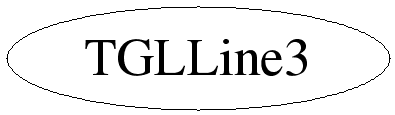# class TGLLine3

```
TGLLine3

3D space, fixed length, line class, with direction / length 'vector',
passing through point 'vertex'. Just wraps a TGLVector3 / TGLVertex3
pair.

```

## Function Members (Methods)

public:
 TGLLine3(const TGLLine3&) TGLLine3(const TGLVertex3& start, const TGLVertex3& end) TGLLine3(const TGLVertex3& start, const TGLVector3& vector) virtual ~TGLLine3() static TClass* Class() void Draw() const const TGLVertex3 End() const virtual TClass* IsA() const TGLLine3& operator=(const TGLLine3&) void Set(const TGLVertex3& start, const TGLVertex3& end) void Set(const TGLVertex3& start, const TGLVector3& vector) virtual void ShowMembers(TMemberInspector& insp, char* parent) const TGLVertex3& Start() const virtual void Streamer(TBuffer& b) void StreamerNVirtual(TBuffer& b) const TGLVector3& Vector() const

## Data Members

private:
 TGLVector3 fVector ! Vector of line from fVertex TGLVertex3 fVertex ! Start vertex of line

## Class Charts## Function documentation

TGLLine3(const TGLVertex3 & start, const TGLVertex3 & end)
``` Construct 3D line running from 'start' to 'end'
```
TGLLine3(const TGLVertex3 & start, const TGLVector3 & vect)
``` Construct 3D line running from 'start', magnitude 'vect'
```

``` Destroy 3D line object
```
void Set(const TGLVertex3 & start, const TGLVertex3 & end)
``` Set 3D line running from 'start' to 'end'
```
void Set(const TGLVertex3 & start, const TGLVector3 & vect)
``` Set 3D line running from start, magnitude 'vect'
```
void Draw()
``` Draw line in current basic GL color. Assume we are in the correct reference
frame
```
TGLLine3(const TGLVertex3 & start, const TGLVertex3 & end)
const TGLVertex3 & Start()
``` Bitwise copy constructor and = operator are fine
Accessors
```
`{ return fVertex; }`
const TGLVertex3 End()
`{ return fVertex + fVector; }`
TGLUtil& operator=(const TGLLine3& )

Author: Richard Maunder 25/05/2005
Last update: root/gl:\$Id: TGLUtil.h 21653 2008-01-11 20:23:15Z matevz \$